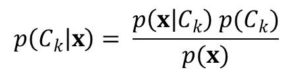# Machine Learning 101 – The Bayes’ Classfier

This is the second lecture on classification. It follow the first one: Introduction to Classification.

Bayes’ Classifier is a classifier that works based on Bayes’ Theorem. It assigns each observation to the most likely class given the values of the measurements.

Also remember that Bayes’ theorem helps us compute conditional probabilities. So a Bayes’ classifier determines the conditional probability for the particular class given the features.

Let’s take the X-ray example.

When an X-ray image of the patient is obtained, the objective is to decide which of the two classes to assign it.  Either C1 or C2.

There for we need to compute the conditional probabilities of the classes C1 and C2 given the image.  Let’s say Ck, where k=1, 2.

The conditional probabilities is given by:In this equation:

P(Ck) is the prior probability for the class Ck. This is because the probability is know prior to taking the X-ray.

P(Ck | x) is the corresponding posterior probability. This is the probability of Ck after taking the X-ray. That is after x has been determined.

If the objective is to reduce the chance of assigning x to the wrong class, then we would choose the class that have the higher posterior probability.

In the case of two-class problem we just discussed, then Bayes’ classifier corresponds to trying to predict  has P(Ck | x) > 0.5. Therefore the corresponding posterior probability would be < 0.5

Since we know that P(C1 | x) + P(C2 | x) = 1.

Bayes’ classifier is know to do pretty good job in classification. However, there are times when misclassification occurs.

We would end this lesson here so in the next lesson, we discuss how to minimize misclassification rate in Bayes’ classifier.

You could read up Basics of Conditional Probability#### kindsonthegenius

Kindson Munonye is currently completing his doctoral program in Software Engineering in Budapest University of Technology and Economics

View all posts by kindsonthegenius →